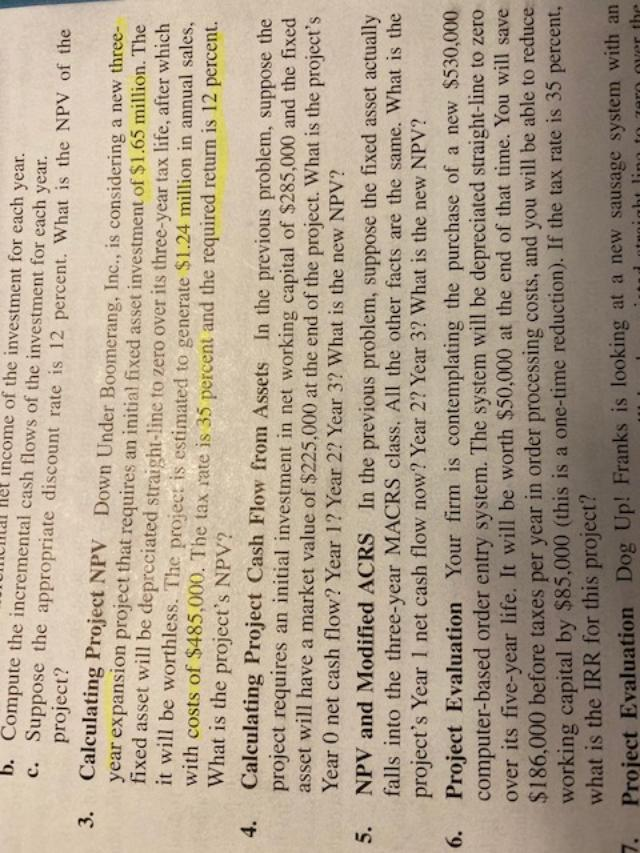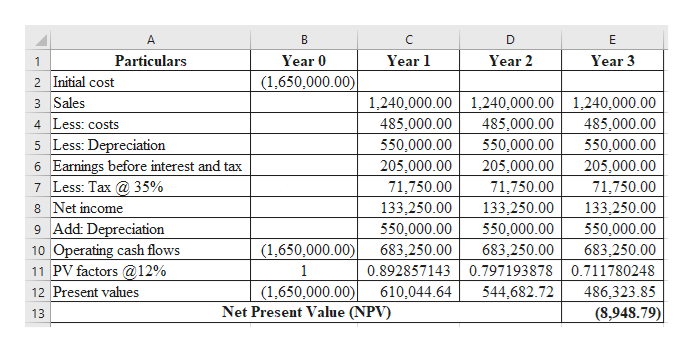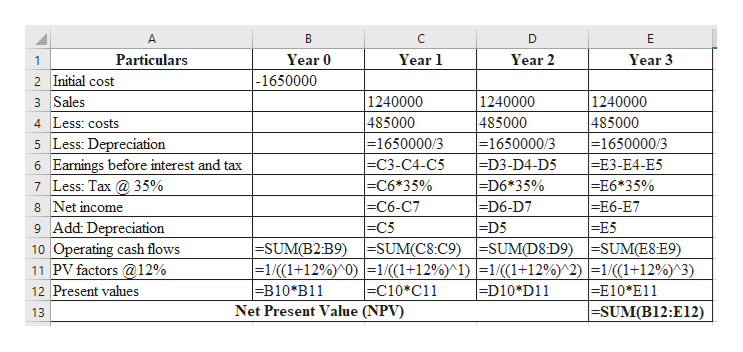# Het income of the investment for each year.b. Compute the incremental cash flows of the investment for each year.c. Suppose the appropriate discount rate is 12 percent. What is the NPV of theproject?3. Calculating Project NPVDown Under Boomerang, Inc., is considering a new three-year expansion project that requires an initial fixed asset investment of \$1.65 million. Thefixed asset will be depreciated straight-line to zero over its three-year tax life, after whichit will be worthless. The project is estimated to generate \$1.24 million in annual sales,with costs of \$485,000. The tax rate is 35 percent and the required return is 12 percent.What is the project's NPV?Calculating Project Cash Flow from Assets In the previous problem, suppose theproject requires an initial investment in net working capital of \$285,000 and the fixedasset will have a market value of \$225,000 at the end of the project. What is the project's4.Year O net cash flow? Year 1? Year 2? Year 3? What is the new NPV?NPV and Modified ACRS In the previous problem, suppose the fixed asset actuallyfalls into the three-year MACRS class. All the other facts are the same. What is theproject's Year 1 net cash flow now? Year 2? Year 3? What is the new NPV?5.Project Evaluationcomputer-based order entry system. The system will be depreciated straight-line to zeroover its five-year life. It will be worth \$50,000 at the end of that time. You will save\$186,000 before taxes per year in order processing costs, and you will be able to reduceworking capital by \$85,000 (this is a one-time reduction). If the tax rate is 35 percent,what is the IRR for this project?6.Your firm is contemplating the purchase of a new \$530,0007.Project Evaluation Dog Up! Franks is looking at a new sausage system with an

Question

Hello, It is a three part question. However, I would like to know how the amount of 122, 265, was calculated in the solution provided for the book. Corporate Finance 11 edition McGraw Hill

See attachmenthelp_outlineImage TranscriptioncloseHet income of the investment for each year. b. Compute the incremental cash flows of the investment for each year. c. Suppose the appropriate discount rate is 12 percent. What is the NPV of the project? 3. Calculating Project NPV Down Under Boomerang, Inc., is considering a new three- year expansion project that requires an initial fixed asset investment of \$1.65 million. The fixed asset will be depreciated straight-line to zero over its three-year tax life, after which it will be worthless. The project is estimated to generate \$1.24 million in annual sales, with costs of \$485,000. The tax rate is 35 percent and the required return is 12 percent. What is the project's NPV? Calculating Project Cash Flow from Assets In the previous problem, suppose the project requires an initial investment in net working capital of \$285,000 and the fixed asset will have a market value of \$225,000 at the end of the project. What is the project's 4. Year O net cash flow? Year 1? Year 2? Year 3? What is the new NPV? NPV and Modified ACRS In the previous problem, suppose the fixed asset actually falls into the three-year MACRS class. All the other facts are the same. What is the project's Year 1 net cash flow now? Year 2? Year 3? What is the new NPV? 5. Project Evaluation computer-based order entry system. The system will be depreciated straight-line to zero over its five-year life. It will be worth \$50,000 at the end of that time. You will save \$186,000 before taxes per year in order processing costs, and you will be able to reduce working capital by \$85,000 (this is a one-time reduction). If the tax rate is 35 percent, what is the IRR for this project? 6. Your firm is contemplating the purchase of a new \$530,000 7. Project Evaluation Dog Up! Franks is looking at a new sausage system with an fullscreen
check_circleExpert Solution
Step 1

3.

Calculate the net present value as follows:help_outlineImage TranscriptioncloseA В C D E Year 1 Particulars Year 0 Year 2 Year 3 1 (1,650,000.00) 2 Initial cost 3 Sales 1,240,000.00 1,240,000.00 1,240,000.00 4 Less: costs 5 Less: Depreciation 6 Earnings before interest and tax 7 Less: Tax 35% 8 Net income 9 Add: Depreciation 10 Operating cash flows 11 PV factors@12% 12 Present values 485,000.00 550,000.00 485,000.00 485,000.00 550,000.00 550,000.00 205,000.00 205,000.00 205,000.00 71,750.00 133,250.00 71,750.00 71,750.00 133,250.00 133,250.00 550,000.00 550,000.00 550,000.00 (1,650,000.00) 683,250.00 683,250.00 683,250.00 0.892857143 0.7971938780.711780248 1 (1,650,000.00) Net Present Value (NPV) 544,682.72 610,044.64 486,323.85 (8,948.79) 13 fullscreen
Step 2

Workings...help_outlineImage TranscriptioncloseA C. D E Year 0 Year 1 Particulars Year 2 Year 3 -1650000 2 Initial cost 3 Sales 1240000 485000 - 1650000/3 =D3-D4-D5 -D6*35% =D6-D7 =D5 |-SUM(B2:B9)-=SUM(C8:C9)=SUM(D8D9) SUM(E8E9) - 1 / ( ( 1 + 12% )^0 ) 1/((1+12%^1)| =1/(1+12%)^2) |=1/(1+12%^3) =D10 * D11 1240000 485000 = 1650000/3 ЕC3-С4-С5 -C6*35% ЕC6-C7 -C5 1240000 485000 - 1650000/3 -E3-E4-E5 -E6*35% =E6-E7 -E5 4 Less: costs 5 Less: Depreciation 6 Earnings before interest and tax 7 Less: Tax35% 8 Net income 9 Add: Depreciation 10 Operating cash flows 11 PV factors12% 12 Present values -C10*C11 Net Present Value (NPV) -B10*B11 =E10*E11 -SUM(B12:E12) 13 fullscreen

### Want to see the full answer?

See Solution

#### Want to see this answer and more?

Solutions are written by subject experts who are available 24/7. Questions are typically answered within 1 hour*

See Solution
*Response times may vary by subject and question
Tagged in